| Table of Contents | Function Reference | Function Finder | R Project | DESCRIBING DATA GRAPHICALLY Introduction The graphical procedures in R are extremely powerful. I'm told there are people who use R not so much for data analysis as for its ability to produce top notch publication quality graphics. I will only scratch the surface of these capabilities here. A later tutorial will fill in a few more of the details. Don't make the mistake of assuming that this tutorial is in any fashion a complete summary of R graphics capabilities. It is a brief overview of how to get a mostly quick-and-dirty graph to visualize your data. R graphics functions can be grouped into three types: High level plotting functions that will create a more or less complete graph, often with axis labels, titles, and so forth. Low level plotting functions that allow additional information to be added to an existing graph, or that allow graphs to be drawn from scratch. Interactive graphics functions that allows the R user to extract information from an existing graph, or to label points and so on. I'm going to do something unusual, and perhaps ill-advised, and cover the low level functions first, so that you will be ready to use them in conjunction with the high level functions when we get to those. If you just want the quick and dirty approach, then skip this first section (for now). Low Level Plotting Functions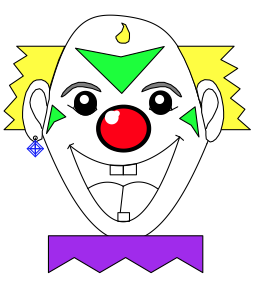For years I told my students, "I can draw anything I want in an R graphics window. I can draw a clown face in an R graphics window if I want to." Finally a student challenged me to do it. It took me the better part of a day to figure out! To conserve space, I'm not going to reproduce the output of every single example in this tutorial. If you have R open and are following along, you can see it on your own screen. High level plotting functions open a graphics device (window) automatically, but the low level functions do not. So to get a graph and some axes to work with, the following command will get us started without actually drawing a graph. `> plot(1:100, 1:100, type="n", xlab="", ylab="")` The plot() function is high level, opening a graphics window and drawing labeled axes, but in this case we've asked it not to plot anything with the type="n" option. Now we have a palette. Let's paint on it. One thing we can do is plot a curve from an algebraic equation. Let's use the equation y = 0.01 x2. `> curve(x^2/100, add=TRUE) # add=T adds the curve to an existing graph` We may want to add some text to the graph, which tells our intended audience just what it is we've plotted. ```> text(x=80, y=50, "This is a graph of") > text(x=80, y=45, "the equation") > text(x=80, y=37, expression(y == frac(1,100) * x^2))``` The text() function takes, first, arguments that give x,y-coordinates at which the text will be centered (and this can take some careful eyeballing or some trial and error), and then it takes quoted text or a mathematical expression. The syntax for the expression() function is an art form in itself (similar to LaTeX), and I have not mastered it, but it can be used to produce some very fancy mathematical expressions. There are also options for controlling font face and size as well as spacing, etc. Next let's draw some points on this curve. `> points(x=c(20, 60, 90), y=c(4, 36, 81), pch=6) # or points(x<-c(20,60,90), y=x^2/100, pch=6)` The first vector gives the x-coordinates of the desired points, the second vector gives the y-coordinates (which can be calculated on the fly), and the "pch=" option gives the point character to use. There are about twenty different point characters to choose from. To see some of them, do this. `> points(x=rep(100,10), y=seq(0,90,10), pch=seq(1,20,2))` You can experiment for yourself to find out what the rest of them look like. Now let's draw a straight line through a couple of those points, say the one at (20, 4) and the one at (90, 81). The draw-a-straight-line function is abline(), and in this case it's arguments are "a=the y-intercept" and "b=the slope" of the desired line. `> abline(a=-18, b=1.1, col="red")` And just to be showy, we made it red with col="red". We can also draw horizontal and vertical lines with this function. ```> abline(h=20, lty=2) # abline(h=20, lty="dashed") also works > abline(v=20, lty=3) # abline(v=20, lty="dotted") also works``` The "lty=" option specifies the line type (1=solid, 2=dashed, 3=dotted). You can also change the color of these lines with col=, and the width of the lines with lwd= options. Try repeating that last command but set lty=1 and lwd=3. We can also draw lines and/or points using the lines() function. ```> lines(x=c(40, 40, 60, 60), y=c(80, 100, 100, 80), type="b") > lines(x=c(40, 60), y=c(80, 80), type="l") # type="lower case L", not "one"``` Once again, the first vector gives the x-coordinates, the second vector the y-coordinates, and the "type=" option tells whether you want just points (type="p"), just lines (type="l"), or both (type="b"). Note: For just lines, use a lower case L. This example shows that type="l" and type="b" behave a bit differently in terms of where the line begins and terminates. Finally, at least as far as this tutorial is concerned, titles and axis labels can be added using the title() function. (NOTE: If you already have axis labels (they are set by default in the plot() function, but we set them to blank by setting xlab="" and ylab=""), you can try a little trick that SOMETIMES works to erase them. Try writing over them in the background color of the graph, in this case, white. This brings up an IMPORTANT POINT. Be careful when you're drawing a complex graph, because it's generally true that, when you make a mistake, you start again! It's advisable to use the script window to write out the commands for a complex graph.) ```> title(main="A Drawing To Put On the Refrigerator!") > title(xlab="This is the x-axis", col.lab="green", cex.lab=1.5)``` This example also gives a little taste of how various options can be used to control colors, fonts, text sizes, and so forth. We'll do more of this in a future tutorial. The col.lab= option sets the color of the label, while the cex.lab= option controls it's size. "cex" stands for character expansion factor, so setting cex.lab=1.5 makes the label 1.5 times it's normal size. And now let's have a look at our masterpiece.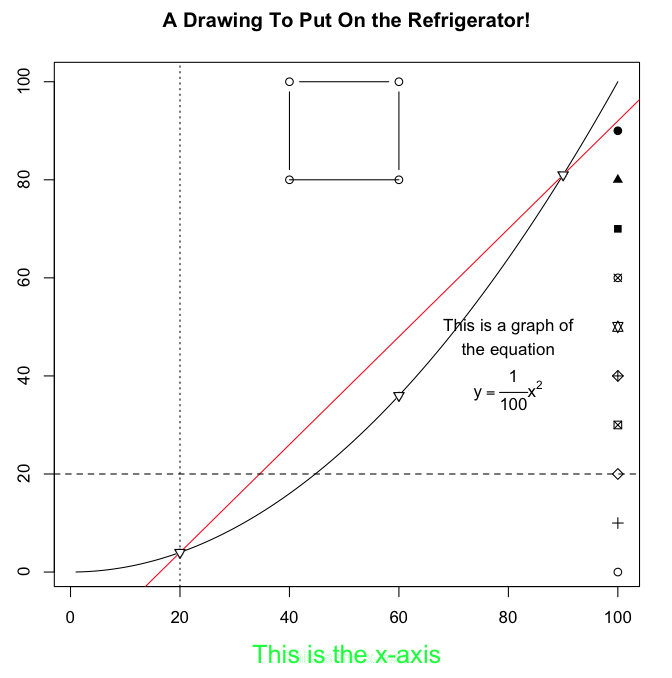Beautiful! Okay, so it's a little first-graderish as R graphics go. There are entire books on R graphics, and I am but a novice! Here is the entire script if you just now have decided you want to see this happen on your own monitor. ```# start copying here plot(1:100, 1:100, type="n", xlab="", ylab="") curve(x^2/100, add=TRUE) text(x=80, y=50, "This is a graph of") text(x=80, y=45, "the equation") text(x=80, y=37, expression(y == frac(1,100) * x^2)) points(x=c(20,60,90), y=c(4,36,81), pch=6) points(x=rep(100,10), y=seq(0,90,10), pch=seq(1,20,2)) abline(a=-18, b=1.1, col="red") abline(h=20, lty=2) abline(v=20, lty=3) lines(x=c(40,40,60,60), y=c(80,100,100,80), type="b") lines(x=c(40,60), y=c(80,80), type="l") title(main="A Drawing To Put On the Refrigerator!") title(xlab="This is the x-axis", col.lab="green", cex.lab=1.5) # stop copying here and paste to your R Console``` You can also paste this into a script window (File > New Document on a Mac, File > New Script in Windows) and experiment with it. Learn by doing! High Level Plotting Functions Usually, we don't want to fuss that much. We just want to see a graph of some data we're examining. If we want to dress it up for publication, THEN we'll worry about the low-level functions and various options. The basic high level plotting function is plot(), and it works differently depending upon what you're asking it to plot. The basic syntax is plot(x, y, ...), where x is a vector of x-coordinates, y is a vector of y-coordinates, and ... represents further refinements and options, as will be illustrated. ```> data(faithful) > attach(faithful) > names(faithful)  "eruptions" "waiting" > plot(x=waiting, y=eruptions) # x is num., y is num., plot is a scatterplot > detach(faithful) > rm(faithful) > > > data(ToothGrowth) > attach(ToothGrowth) > names(ToothGrowth)  "len" "supp" "dose" > plot(x=supp, y=len) # x is factor, y is num., plot is boxplots > plot(x=factor(dose), y=len) # coercing dose to a factor > detach(ToothGrowth) > rm(ToothGrowth) > > > data(sunspots) > class(sunspots)  "ts" > plot(sunspots) # x is time series, y missing, plot is a > rm(sunspots) # time-series plot > > > data(UCBAdmissions) > class(UCBAdmissions)  "table" > plot(UCBAdmissions) # x is table, y missing, plot is a mosaic plot > rm(UCBAdmissions) > > > data(mtcars) > str(mtcars) 'data.frame': 32 obs. of 11 variables: \$ mpg : num 21 21 22.8 21.4 18.7 18.1 14.3 24.4 22.8 19.2 ... \$ cyl : num 6 6 4 6 8 6 8 4 4 6 ... \$ disp: num 160 160 108 258 360 ... \$ hp : num 110 110 93 110 175 105 245 62 95 123 ... \$ drat: num 3.9 3.9 3.85 3.08 3.15 2.76 3.21 3.69 3.92 3.92 ... \$ wt : num 2.62 2.88 2.32 3.21 3.44 ... \$ qsec: num 16.5 17.0 18.6 19.4 17.0 ... \$ vs : num 0 0 1 1 0 1 0 1 1 1 ... \$ am : num 1 1 1 0 0 0 0 0 0 0 ... \$ gear: num 4 4 4 3 3 3 3 4 4 4 ... \$ carb: num 4 4 1 1 2 1 4 2 2 4 ... > plot(mtcars) # x is dataframe of num. vars., y missing, > rm(mtcars) # plot is a scatterplot matrix >```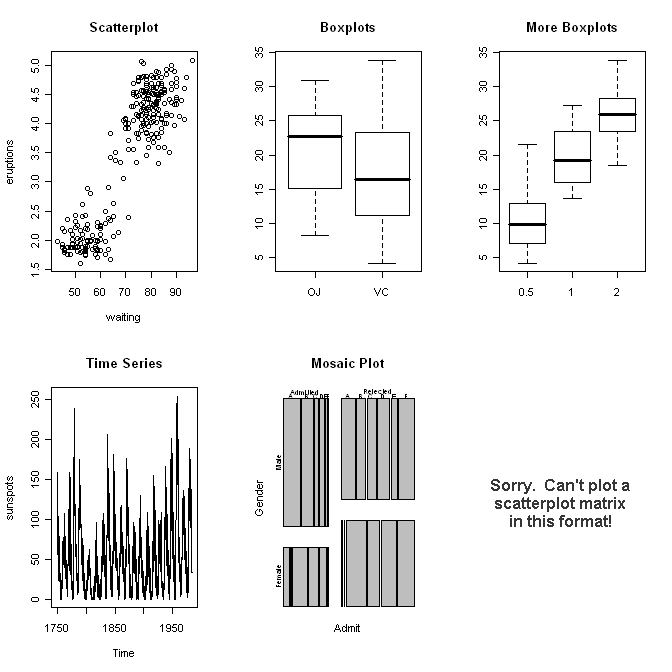And so on. I think I've made my point. As we go through the individual data analyses in future tutorials, we will see these various plots again, and we will dress them up a bit. So for now, let me just illustrate a few other things R can do. Note: Plotting can also be done with a formula interface: plot(y ~ x). Pie Charts and Bar Graphs When a single categorical variable is being graphed, the customary way is to use a pie chart or a bar graph. Statisticians are somewhat biased against pie charts, and I suppose for good reason, but I'll illustrate them anyway, just in case you have a hankerin' to flout good statistical practice. The data set UCBAdmissions, which we were using above, is the Berkeley admissions data we used in a different form in a previous tutorial. The data set is a 3-D table, and we need a 1-D table to illustrate a basic piechart and barplot, so... ```> margin.table(UCBAdmissions, 3) # Collapse over dimensions 1 and 2. Dept A B C D E F 933 585 918 792 584 714 > margin.table(UCBAdmissions,3) -> Department > pie(Department) > barplot(Department, xlab="Department", ylab="frequency")```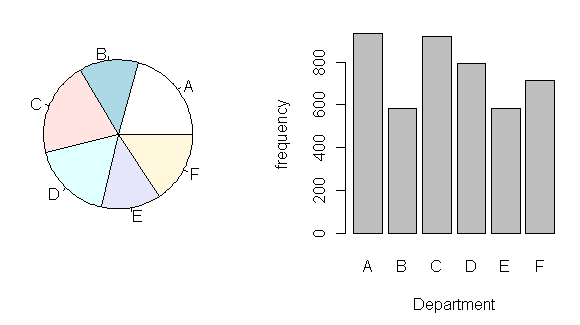I think you can see why the bar graph is preferred. It's much easier to read. The pie() function in R is limited because, as I mentioned above, many statisticians (including the R folks) consider pie charts to be poor statistical practice. However, if you want something flashy like a 3D exploded pie chart, you can get it by installing an optional graphics package called "plotrix", which contains a function called pie3D(), which has an "explode" option. It just goes to show, if you want it, someone has probably written an R package that will do it! To see an example of an exploded pie chart produced with this package, try this link. In fact, I recommend the plotrix package if you want some useful extensions to the basic R graphics capabilities. If you want to look at two categorical variables at once, a stacked barplot, or better yet, a side-by-side barplot is usually the way to go. ```> margin.table(UCBAdmissions, c(1,3)) -> Admit.by.Dept > barplot(Admit.by.Dept) > barplot(Admit.by.Dept, beside=T, ylim=c(0,1000), legend=T, + main="Admissions by Department")```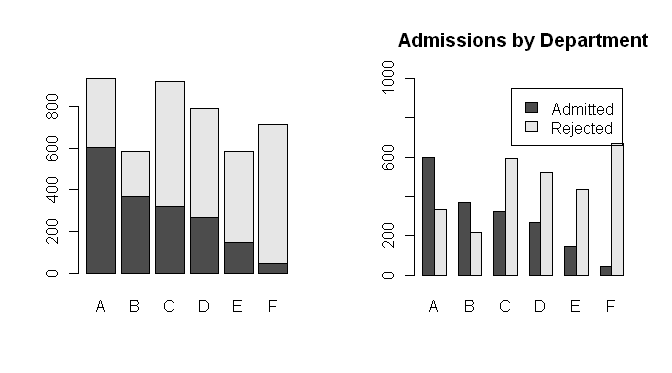Notice a stacked barplot is the default for some odd reason. To change that, set the "beside=" option to TRUE. Also, I dressed up the second barplot a bit by adding a main title, and by changing the limits on the y-axis to (almost!) make enough room for a legend. I need to adjust the font size a bit in the legend, and maybe change its location, but that's a future tutorial! Histograms When you have one numeric variable to look at, a histogram is appropriate. I'll use the "faithful" data set again to illustrate. ```> data(faithful) # This is optional. > attach(faithful) > hist(waiting)```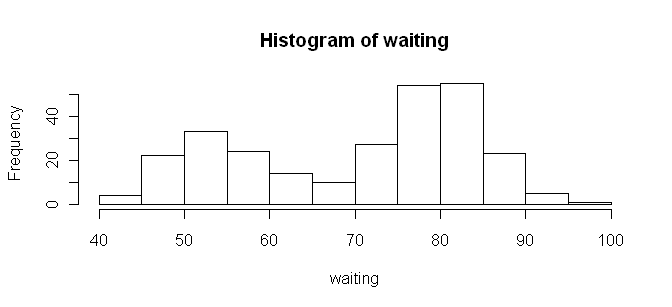It doesn't get much more straightforward than that! And by the way, in case you're wondering, I resized the graphic by resizing the graphics device window before saving it. There are better ways, but that works pretty well in a pinch. If you want more or fewer bars, you can refine your plot by using the "breaks=" option and defining your own breakpoints. ```> range(waiting)  43 96 > hist(waiting, breaks=seq(from=40, to=100, by=10))``` By default, R includes the right limit (right side of the bar) but not the left limit in the intervals. Usually, I prefer it the other way around, so I change it with the "right=" option, which by default is TRUE. `> hist(waiting, breaks=seq(40,100,10), right=F)` There are many, many other options as well, which you can examine by looking at the help page for this function: type ?hist. R also incorporates many functions for data smoothing, including kernel density smoothing of histograms. If you'd rather see a smooth curve than a boxy histogram, it can be done as follows. ```> plot(density(waiting)) > # Or, getting fancier... > hist(waiting, prob=T) > lines(density(waiting)) > detach(faithful)```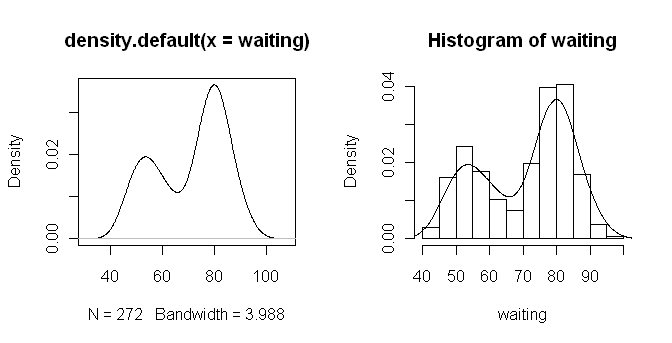The density() function does kernel density smoothing, which can be refined by adjusting the options of the function. To plot the smoothed curve on top of a histogram, set the "prob=" option to TRUE inside the hist() function. This plots densities rather than frequencies. Also, use lines() rather than plot() to draw the smoothed curve. This low level graphics function will add the smoothed curve to the histogram rather than drawing a new plot and thereby erasing the histogram. Numerical Summaries by Groups When you have a numerical variable indexed by a categorical variable or factor, you might want a group-by-group summary in graphical form. The primary way R offers to achieve this is side-by-side boxplots. ```> data(chickwts) # Weight gain by type of diet. > str(chickwts) 'data.frame': 71 obs. of 2 variables: \$ weight: num 179 160 136 227 217 168 108 124 143 140 ... \$ feed : Factor w/ 6 levels "casein","horsebean",..: 2 2 2 2 2 2 2 2 2 2 ... > attach(chickwts) > plot(feed, weight) # boxplot(weight ~ feed) will also work > title(main="Body Weight of Chicks by Type of Diet")```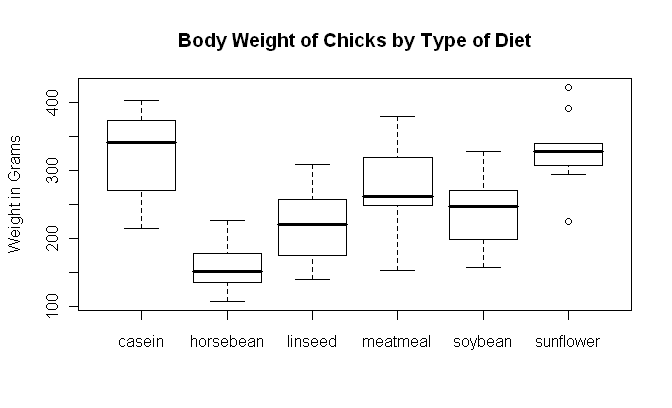One somewhat annoying thing about boxplots is that they don't display means. If you're doing a parametric analysis such as t-test or ANOVA, those are tests on means, so your graphic ought to display means. You can add means by using the low-level points() function. (Watch your screen, I won't reproduce this here.) ```> means = tapply(weight, feed, mean) > points(x=1:6, y=means, pch=16) # pch=16 is a filled circle > detach(chickwts)``` The function boxplot(), which takes a formula interface, can also be used. Here is the example copied and pasted off the "chickwts" help page. ```> boxplot(weight ~ feed, data = chickwts, col = "lightgray", + varwidth = TRUE, notch = TRUE, main = "chickwt data", + ylab = "Weight at six weeks (gm)") Warning message: In bxp(list(stats = c(216, 271.5, 342, 373.5, 404, 108, 136, 151.5, : some notches went outside hinges ('box'): maybe set notch=FALSE``` Notice that several options are set, including an option to color the boxes, the "varwidth=" option, which sets the width of the box according to the sample size, the "notch=" option, which gives a confidence interval around the median, and options to print a main title and y-axis label. The procedure generated a warning message, which you will understand when you look at the graphic (which I have not reproduced here). Scatterplots For examining the relationship between two numerical variables, you can't beat a scatterplot. R has several functions for producing them, two of which will be demonstrated here. ```> data(mammals, package="MASS") > str(mammals) 'data.frame': 62 obs. of 2 variables: \$ body : num 3.38 0.48 1.35 465.00 36.33 ... \$ brain: num 44.5 15.5 8.1 423.0 119.5 ... > attach(mammals) > plot(log(body), log(brain)) # plot(x=body, y=brain, log="xy") is similar (try it) > scatter.smooth(log(body), log(brain)) > detach(mammals)```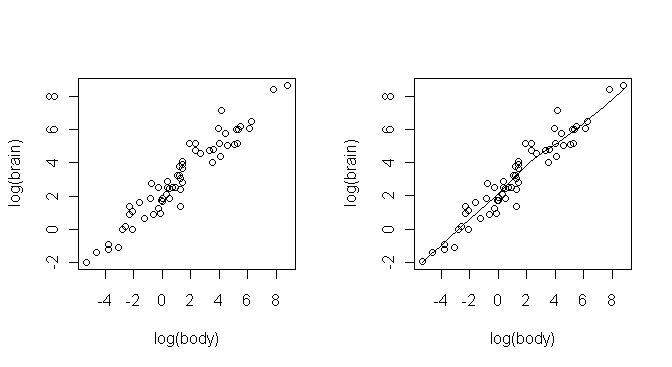Some explanations are in order. First, I didn't want to attach the MASS package to the search path, so I used an option when I copied the "mammals" data frame that told R to look for it there. The data frame contains brain and body weights from 62 species of land mammals. Second, to produce a linear plot, I had to do a log transform on both variables, and I did that "on the fly." Third, the two functions produced the same scatterplot, but the scatter.smooth() function also plots a smoothed, nonparametric regression line on the plot. This line is computed using the loess technique and is called the "loess line" (locally weighted scatterplot smoothing, sometimes also called "lowess", although I understand some sources use the two acronyms differently). Both functions have options that allow the plots to be modified in several ways. Interacting With Plots R supplies several functions that allow you to interact with the graphics window, including functions that allow you to identify and label points on the graph. See the help pages for the locator() and identify() functions for details. I'll discuss these briefly in a later tutorial. Remember to clean up your workspace! revised 2016 January 31 | Table of Contents | Function Reference | Function Finder | R Project |